# GSEB Solutions Class 11 Maths Chapter 8 Binomial Theorem Miscellaneous Exercise

Gujarat Board GSEB Textbook Solutions Class 11 Maths Chapter 8 Binomial Theorem Miscellaneous Exercise Textbook Questions and Answers.

## Gujarat Board Textbook Solutions Class 11 Maths Chapter 8 Binomial Theorem Miscellaneous Exercise

Question 1.
Find a, b and n in the expansion of (a + b)n, if the first three terms of the expansion are 729, 7290 and 30375 respectively.
Solution:
T1 of (a + b)n = an = 729 ……………….. (1)
T2 of (a + b)n = nC1an-1b = 7290 ……………… (2)
T3 of (a + b)n = nC2an-2b2 = 30375 …………………. (3)
Dividing (1) by (2), we get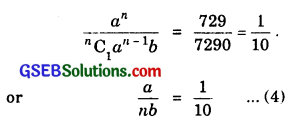Dividing (2) by (3), we get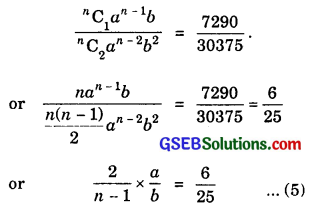Dividing (4) by 5, we get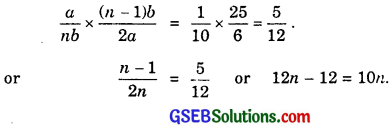or 2n = 12 ⇒ n = 6.
Also, putting n = 6 in (1), we get a6 = 729 ⇒ a = 3
Putting n = 6 and a = 3 in (4), we get
$$\frac{3}{6b}$$ = $$\frac{1}{10}$$.
∴ b = $$\frac{3×10}{6}$$ = 5.
Thus, a = 3, b = 5, n = 6.Question 2.
Find a, if the coefficients of x2 and x3 in the expan sion of (3 + ax)9 are equal.
Solution:
General Term = Tr+1 = 9Cr.39-rarxr.
Putting r = 2, we get
Coefficient of x2 = 9C239-2.a2 = $$\frac{9×8}{2}$$.37a2 = 4.39a2 …………………. (1)
Putting r = 3, we get
Coefficient of x3 = 9C339-3a3 = $$\frac{9×8×7}{6}$$ × 36.a3
= 4 × 7 × 37.a3
Equating (1) and (2), we get
4.39.a2 = 4 × 7 × 37a3, we get
or 32 = 7a ⇒ a = $$\frac{9}{7}$$.

Question 3.
Find the coefficient of x5 in the expansion of the product (1 + 2x)6(1 – x)7.
Solution:
(1 + 2x)6 = 6C0.1 + 6C1(2x) + 6C2(2x)2 +
6C3(2x)3 + 6C4(2x)4 + 6C5(2x)5 + 6C6(2x)6
= 1 + 12x + 60x2 + 20 × 8x3
+ 15 × 16x4 + 6 × 32x6 + 64x5
= 1 + 12x + 60x2 + 160x3
+ 240x4 + 192x5 + 64x5 …………………. (1)
(1 – x)7 = 1 – 7C1x + 7C2x2
7C3x3 + 7C4x47C5x5 + 7C6x67C7x7
= 1 – 7x + 21x2 – 35x3 + 35x4 – 21x5 + 7x6 – x7 …………………… (2)
Multiplying (1) and (2) and collecting the coefficients of x5, we get
Coefficient of x5 in the product (1 + 2x)0(1 – y)7
= 1 × (- 21) + 12 × 35 + 60 × (- 35) + 160 × 21 + 240 × (- 7) + 192 × 1
= – 21 + 420 – 2100 + 3360 – 1680 + 192 = 171.Question 4.
If a and b are distinct integers, prove that a – b is a factor of an – bn, whenever n is a positive integer?
Solution:
Now a = a + b – b = b + (a – b)
an = b + (a – b)n
= bn + nC1an-1(a – b) + nC2bn-2(a – b)2 + ………………… + (a – b)n
or an – bn = nC1bn-1(a – b) + nC2(a – b)2 + ……………. + (a – b)n
= (a – b) = (a – b)[nC1bn-1 + nC2bn-2(a – b) + ……………. + (a – b)x-1]
Thus, (a – b) is a factor of (an – bn).

Question 5.
Evaluate ($$\sqrt{3}$$ + $$\sqrt{2}$$)6 – ($$\sqrt{3}$$ – $$\sqrt{2}$$)6.
Solution:
($$\sqrt{3}$$ + $$\sqrt{2}$$)6 = ($$\sqrt{3}$$)6 + 6C1($$\sqrt{3}$$)5($$\sqrt{2}$$)
+ 6C2($$\sqrt{3}$$)4($$\sqrt{2}$$)2 + 6C5($$\sqrt{3}$$)($$\sqrt{2}$$)5 + ($$\sqrt{2}$$)5 ………………. (1)
($$\sqrt{3}$$ – $$\sqrt{2}$$)6 = ($$\sqrt{3}$$)66C1($$\sqrt{3}$$)5($$\sqrt{2}$$) + 6C2($$\sqrt{3}$$)4($$\sqrt{2}$$)4
6C5($$\sqrt{3}$$)($$\sqrt{2}$$)5 + ($$\sqrt{2}$$)5 ………………. (2)
Subtracting (2) from (1), we get
($$\sqrt{3}$$ + $$\sqrt{2}$$)6 – ($$\sqrt{3}$$ – $$\sqrt{2}$$)6
= 2[6C1($$\sqrt{3}$$)5($$\sqrt{2}$$) + 6C3($$\sqrt{3}$$)3 + 6C5($$\sqrt{3}$$)($$\sqrt{2}$$)5]
= 2[6.35/2.21/2 = 20.33/223/2 + 6.31/2.25/2]
= 2.31/2.21/2[6.32 + 20.33/223/2 + 6.31/2.25/2]
= 2.31/2.21/2[6.32 + 20.3.2 + 6.22]
= 2$$\sqrt{6}$$ × 198 = 396$$\sqrt{6}$$.Question 6.
Find the value of (a2 + $$\sqrt{a^{2}-1}$$)4 + (a2 – $$\sqrt{a^{2}-1}$$)4.
Solution:
Put a2 = x, $$\sqrt{a^{2}-1}$$ = y.
∴ (x + y)4 = x4 + 4C1x3y + 4C2x2y2 + 4C3xy3 + 4C4y4 ………………… (1)
(x – y)4 = x44C1x3y + 4C2x2y24C3xy3 + 4C4y4 ………………. (2)
Adding (1) and (2), we get
(x + y)4 + (x – y)4 = 2[x4 + 4C2x2y2 + 4C4y4] = 2[x4 + 6x2y2 + y4].
∴ (a2 + $$\sqrt{a^{2}-1}$$4 + (a2 – $$\sqrt{a^{2}-1}$$)4
= 2[a2)4 + 6(a2)2($$\sqrt{a^{2}-1}$$)2 + ($$\sqrt{a^{2}-1}$$)4].
= 2[a8 + 6a4(a2 – 1) + (a2 – 1)2]
= 2[a8 + 6a4(a2 – 1) + a4 – 2a2 + 1]
= 2[a8 + 6a6 – 5a4 – 2a2 + 1].

Question 7.
Find an approximation of (0.99)5, using the first three terms of its expansion?
Solution:
(0.99)5 = (1 – 0.01)5
= 1 – 5C1 × (0.01) + 5C2 × (0.01)2 ……
= 1 – 0.05 + 10 × 0.0001 ……
= 1.001 – 0.05
= 0.951.Question 8.
Find x, if the ratio of the fifth term from the beginning to the fifth term from the end in the expansion of ($$\sqrt{2}+\frac{1}{\sqrt{3}}$$)n is $$\sqrt{6}$$ : 1.
Solution: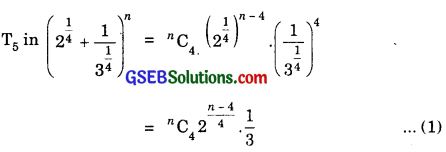Total number of terms = n + 1.
Fifth term from the end
= [(n + 1) – 5 + 1]th term from the beginning
= (n – 3)th term from the beginning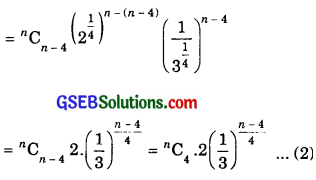Dividing (1) by (2), we get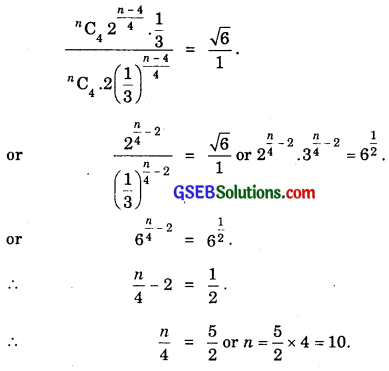Question 9.
Expand using Binomial theorem (1 + $$\frac{x}{2}$$ – $$\frac{2}{x}$$)4, x ≠ 0.
Solution: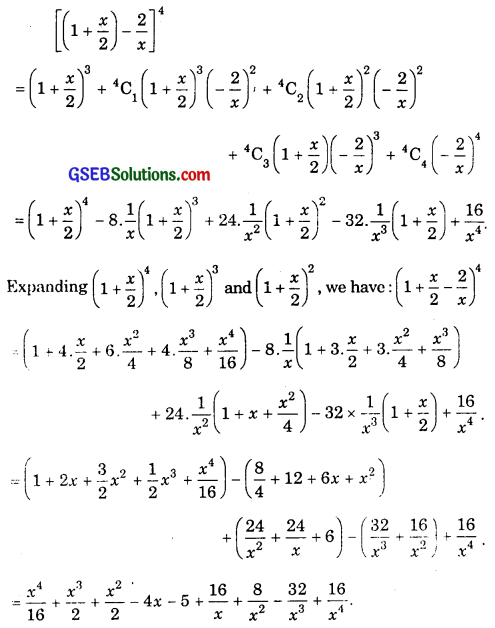Question 10.
Find the expansion of (3x2 – 2ax + 3a2)3, using Binomial theorem?
Solution:
[3x2 – a(2x – 3a)]3
= (3x2)33C1(a2)2.a(2x – 3a) + 3C2(3x2)a2 – a3(2x – 3a)3.
= 27x6 – 27x4ax – 3a) + 9x2a2(4x2 – 12ax + 9a2)
– a3(8x3 – 3.4x2.3a + 3.2x9a2 – 27a3).
= 27x6 – 54x5a + 81a2x4 + 36a2x4 – 108a3x3
+ 81a4x2 – 8a3x3 + 36a4x2 – 54a5x + 27a6.
= 27x6 – 54ax5 + 117a2x4 – 116a3x3 + 117a4x2 – 54a5x + 27a6.